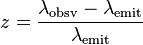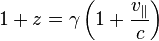# Doppler Shift Light from another galaxy

When we analyze the light coming from a distant galaxy, we find a particular absorption line with a wavelength of 1118 nm. This same absorption line in light from the sun has a wavelength of 625 nm is this galaxy moving towards us or away from us?

Calculate the magnitude of the velocity of the galaxy relative to us.

I've tried all what I think are the Doppler equations for light, but can't seem to get the right answer.

When I plug in the values to λr = λc/(c − vr), I get 1.32198284 * 10^8 m / s, which the online homework says is wrong.

Bave you tried:and?

gneill
Mentor
If the classical approach (as you have used) returns a value for the velocity that is a significant fraction of the speed of light (in your case about 44% of c), then you'll want to employ the Relativistic Doppler Shift instead.

λ_s/λ_r = sqrt((1 + beta)/(1 - beta))

where: beta = v/c

This can be rearranged as:

v/c = (λ_s2 - λ_r2)/(λ_s2 + λ_r2)